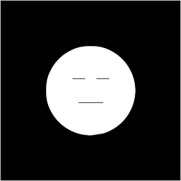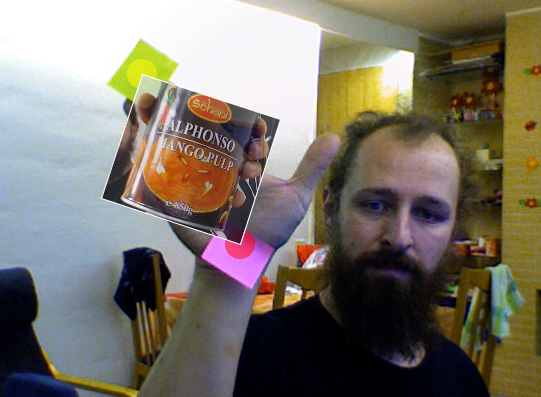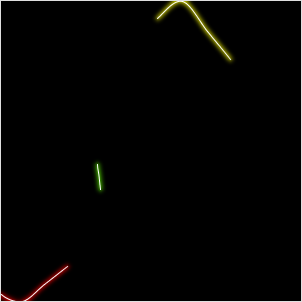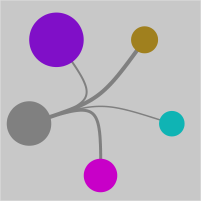## ColorMatcher Processing lib

a while ago i wrote a processing sketch that allows to match some colors in a live video image.

now i translated the code into a processing library and made a project-page for it. The libray is released under the LGPL.

## Processing monster

This time i haven't found a monster - i made one. in processing.## Fun with sine and cosine

I just played a bit with sine and cosine and got a very nice picture. the code takes some time to run, so i post a pregenerated image this time.

``````float r;
void setup() {
size(600,600);
smooth();
r = max( width/2, height/2) * 0.8;
noLoop();
}
void draw() {
background(0);
stroke( 255, 2 );
strokeWeight(2);
lines( 4 );
filter( BLUR, 4 );
stroke( 255, 6 );
strokeWeight(1);
lines( 2);
}

void lines( float factor ) {
for ( int j = 0; j < 360 * 10 * factor; j++ ) {
float i = j/factor;
line( width/2 - r * cos( radians( i / 2.1 )),
height/2 - r * sin( radians( i  )),
width/2 - r * sin( radians ( i / 1.01  )),
height/2 - r * cos( radians( 180 - i ))
);
}
}
``````

## Linedemo in processing.js

Todays usless processing-sketch is written in a processing dialect written in java-script. The demo shows a rotating line and uses the canvas element. So if you can't see anything try another browser.

## Touchless Multitouch in Processing

update (23.11.2008): i made a processing library out of the codeexample above - guru

Today i read about touchless an opensource sdk microsoft has released. it enables you to track objects using a simple webcam to create apps similar to a multitouch display.

then i spent about 20 minutes coding a processing sketch that does roughly the same :-)

as you can see in the screenshot i cant affort the same cool toys the microsoft coder has in the demo video, so i had to use some post-its instead

below is the complete sourcecode of the app. I didn't export it as an applet this time, because the applet couldn't use the quicktime api. It also only works on mac and windows this time, because processing uses the quicktime api. on linux the gstreamer based replacement from codeanticode can be used.

when the programm starts hold up 2 different colored items (use colors that do not appear in the background) then click on the first color with the left mousebutton and on the second color using the right mousebutton. and then enjoy the "minority report"-experience zooming and rotating the image :-)``````import processing.video.*;
import java.util.*;

Capture video;
PImage img;

void setup() {
size( 640, 480 );
video = new Capture( this, width, height, 30 );
noStroke();
smooth();
}

int searchColor1 = color( 128, 255, 0 );
int searchColor2 = color( 255, 0, 0 );

Point e1 = new Point( 0, 0 );
Point e2 = new Point( 640, 480);

boolean s1, s2;

void draw() {
if ( video.available()) {
pushMatrix();
scale(-1,1);
image( video, -width, 0, width, height );
popMatrix();

int idx = 0;

ArrayList p1 = new ArrayList();
ArrayList p2 = new ArrayList();
for ( int y = 0; y < video.height ; y++ ) {
for ( int x = video.width; x >0; x-- ) {
if ( match( searchColor1, video.pixels[idx] )) {
p1.add( new Point( x, y ));
//fill( 255, 255, 0, 128 );
//ellipse( x, y, 10, 10 );
} else if (match( searchColor2, video.pixels[idx] )){
p2.add( new Point( x, y ));
//fill( 255, 0, 0, 128 );
//ellipse( x, y, 10, 10 );
}
idx ++;
}
}

noStroke();
if (p1.size() > 0) e1 = avg( p1 );
if (p2.size() > 0) e2 = avg( p2 );

if (s1) {
fill( 255, 255, 0, 128 );
ellipse( e1.x, e1.y, 30, 30 );
}
if ( s2 ) {
fill( 255, 0, 0, 128 );
ellipse( e2.x, e2.y, 30, 30 );
}

if (s1 && s2 ) {
pushMatrix();
translate( e1.x, e1.y );
int dx = e2.x - e1.x;
int dy = e2.y - e1.y;
rotate( - atan2( e2.x -  + e1.x, e2.y - e1.y) + atan2( img.width, img.height));
float zoom = sqrt ( dx * dx + dy * dy ) /
sqrt(img.width * img.width + img.height * img.height);
scale(zoom, zoom );

tint( 255, 200 );

image( img, 0, 0 );
strokeWeight( 3 );
stroke( 255 );
noFill();
rect( 0,0,img.width, img.height );
noTint();
popMatrix();
}
}
}

boolean match( int c1, int c2 ) {
int limit = 20;
int sr = c1 >> 16 & 0xFF;
int sg = c1 >> 8 & 0xFF;
int sb = c1 & 0xFF;

int cr = c2 >> 16 & 0xFF;
int cg = c2 >> 8 & 0xFF;
int cb = c2 & 0xFF;

return cr > sr - limit && cr < sr + limit &&
cg > sg - limit && cg < sg + limit &&
cb > sb - limit && cb < sb + limit;
}

void mousePressed() {
if (mouseButton == LEFT) {
searchColor1 = get( mouseX, mouseY );
s1 = true;
}
if (mouseButton == RIGHT) {
searchColor2 = get( mouseX, mouseY );
s2 = true;
}
}

void keyPressed() {
s1 = false;
s2 = false;
}

Point avg( ArrayList l ) {
if (l.size() == 0) {
return new Point( 0, 0 );
}
int x = 0;
int y = 0;
for( Iterator i = l.iterator(); i.hasNext(); ) {
Point p = (Point)i.next();
x += p.x;
y += p.y;
}
return new Point( x  / l.size(), y / l.size());
}

public class Point {
int x;
int y;

Point( int x, int y ) {
this.x = x;
this.y = y;
}
}
``````

## Neonworms

I made another usless little processing-sketch. This time staring some neonworms## Voting trend visualisation

After every election, there is a poll resulting in a voter trend analysis. i always liked the graphs they made from the data on tv. This time i desided to make my own voter trend visualisation in processing.

i didn't know if i would be permitted to use actual data, so i used random() to generate it.i wanted to test the "noLoop()" function from processing, so i did a digital version of them